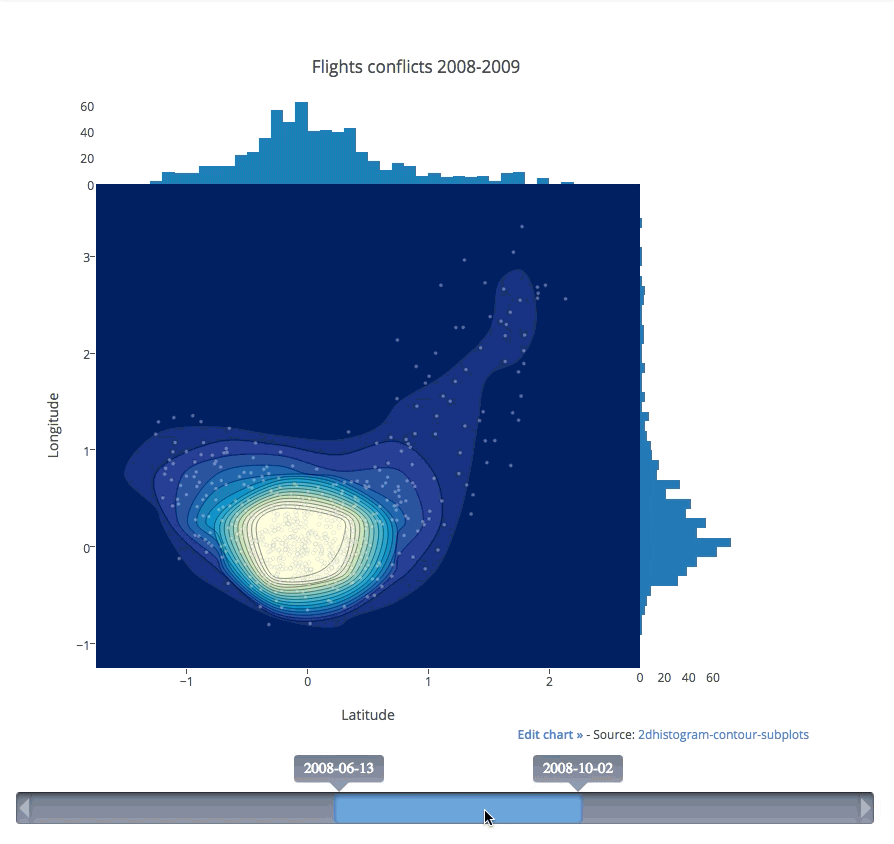# 2d Density Plots in Python/v3

How to make a 2d density plot in python. Examples of density plots with kernel density estimations, custom color-scales, and smoothing.

See our Version 4 Migration Guide for information about how to upgrade.

#### New to Plotly?¶

Plotly's Python library is free and open source! Get started by dowloading the client and reading the primer.
You can set up Plotly to work in online or offline mode, or in jupyter notebooks.
We also have a quick-reference cheatsheet (new!) to help you get started!

#### Version Check¶

Note: 2D Density Plots are available in version 2.0.0+
Run pip install plotly --upgrade to update your Plotly version

In :
import plotly
plotly.__version__

Out:
'2.0.2'

#### 2D Histogram Contour Plot with Histogram Subplots¶

In :
import plotly.plotly as py
import plotly.figure_factory as ff

import numpy as np

t = np.linspace(-1, 1.2, 2000)
x = (t**3) + (0.3 * np.random.randn(2000))
y = (t**6) + (0.3 * np.random.randn(2000))

colorscale = ['#7A4579', '#D56073', 'rgb(236,158,105)', (1, 1, 0.2), (0.98,0.98,0.98)]

fig = ff.create_2d_density(
x, y, colorscale=colorscale,
hist_color='rgb(255, 237, 222)', point_size=3
)

py.iplot(fig, filename='histogram_subplots')

Out:

#### 2D Histogram Contour Plot with Slider Control¶

Add slider controls to 2d-density-plot plots with the postMessage API.

See the code on JSFiddle.

Watch the 5 second video of how it works:#### Reference¶

In :
help(ff.create_2d_density)

Help on function create_2d_density in module plotly.figure_factory._2d_density:

create_2d_density(x, y, colorscale='Earth', ncontours=20, hist_color=(0, 0, 0.5), point_color=(0, 0, 0.5), point_size=2, title='2D Density Plot', height=600, width=600)
Returns figure for a 2D density plot

:param (list|array) x: x-axis data for plot generation
:param (list|array) y: y-axis data for plot generation
:param (str|tuple|list) colorscale: either a plotly scale name, an rgb
or hex color, a color tuple or a list or tuple of colors. An rgb
color is of the form 'rgb(x, y, z)' where x, y, z belong to the
interval [0, 255] and a color tuple is a tuple of the form
(a, b, c) where a, b and c belong to [0, 1]. If colormap is a
list, it must contain the valid color types aforementioned as its
members.
:param (int) ncontours: the number of 2D contours to draw on the plot
:param (str) hist_color: the color of the plotted histograms
:param (str) point_color: the color of the scatter points
:param (str) point_size: the color of the scatter points
:param (str) title: set the title for the plot
:param (float) height: the height of the chart
:param (float) width: the width of the chart

Example 1: Simple 2D Density Plot

import plotly.plotly as py
from plotly.figure_factory create_2d_density

import numpy as np

# Make data points
t = np.linspace(-1,1.2,2000)
x = (t**3)+(0.3*np.random.randn(2000))
y = (t**6)+(0.3*np.random.randn(2000))

# Create a figure
fig = create_2D_density(x, y)

# Plot the data
py.iplot(fig, filename='simple-2d-density')


Example 2: Using Parameters

import plotly.plotly as py
from plotly.figure_factory create_2d_density

import numpy as np

# Make data points
t = np.linspace(-1,1.2,2000)
x = (t**3)+(0.3*np.random.randn(2000))
y = (t**6)+(0.3*np.random.randn(2000))

# Create custom colorscale
colorscale = ['#7A4579', '#D56073', 'rgb(236,158,105)',
(1, 1, 0.2), (0.98,0.98,0.98)]

# Create a figure
fig = create_2D_density(
x, y, colorscale=colorscale,
hist_color='rgb(255, 237, 222)', point_size=3)

# Plot the data
py.iplot(fig, filename='use-parameters')